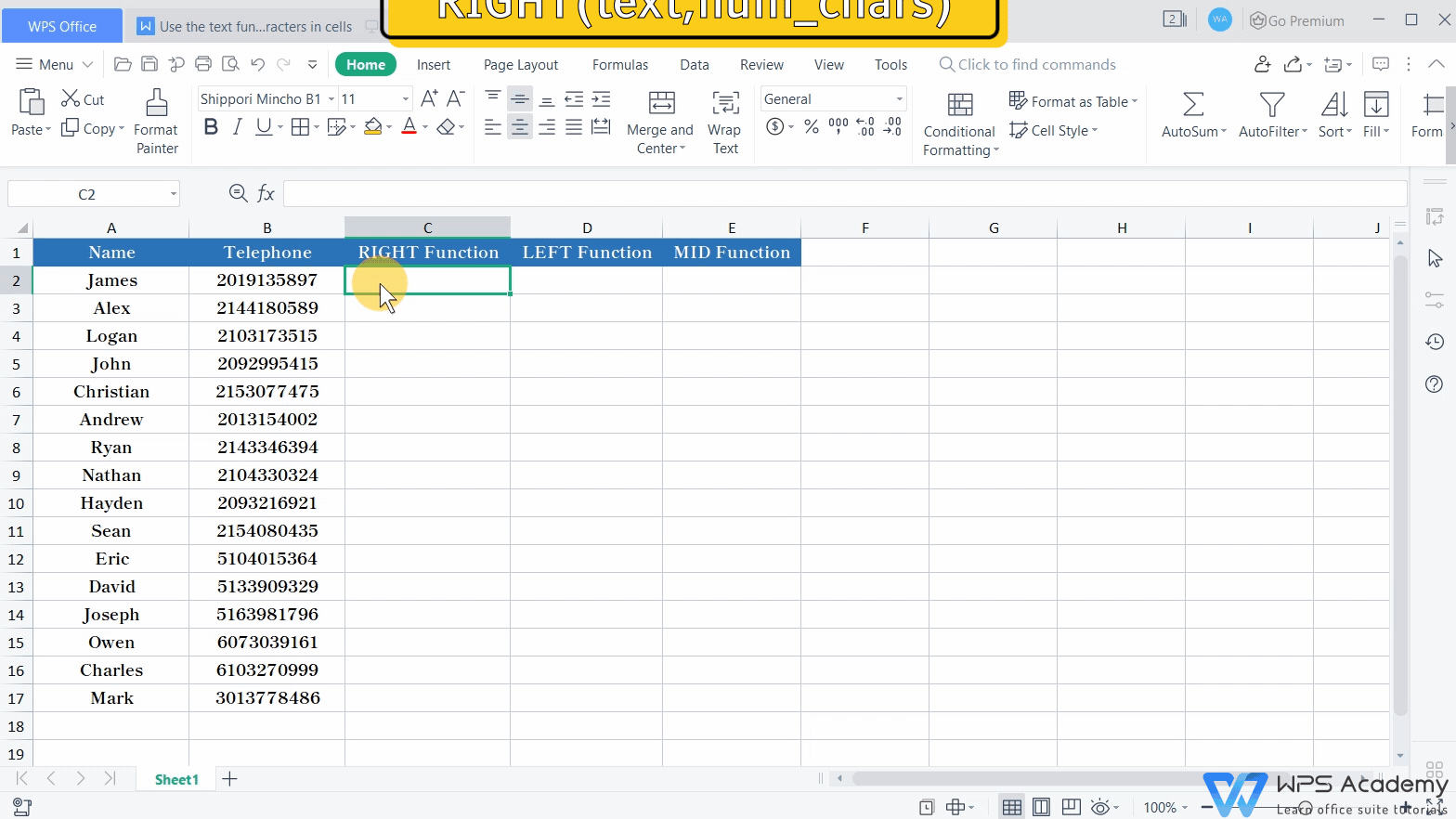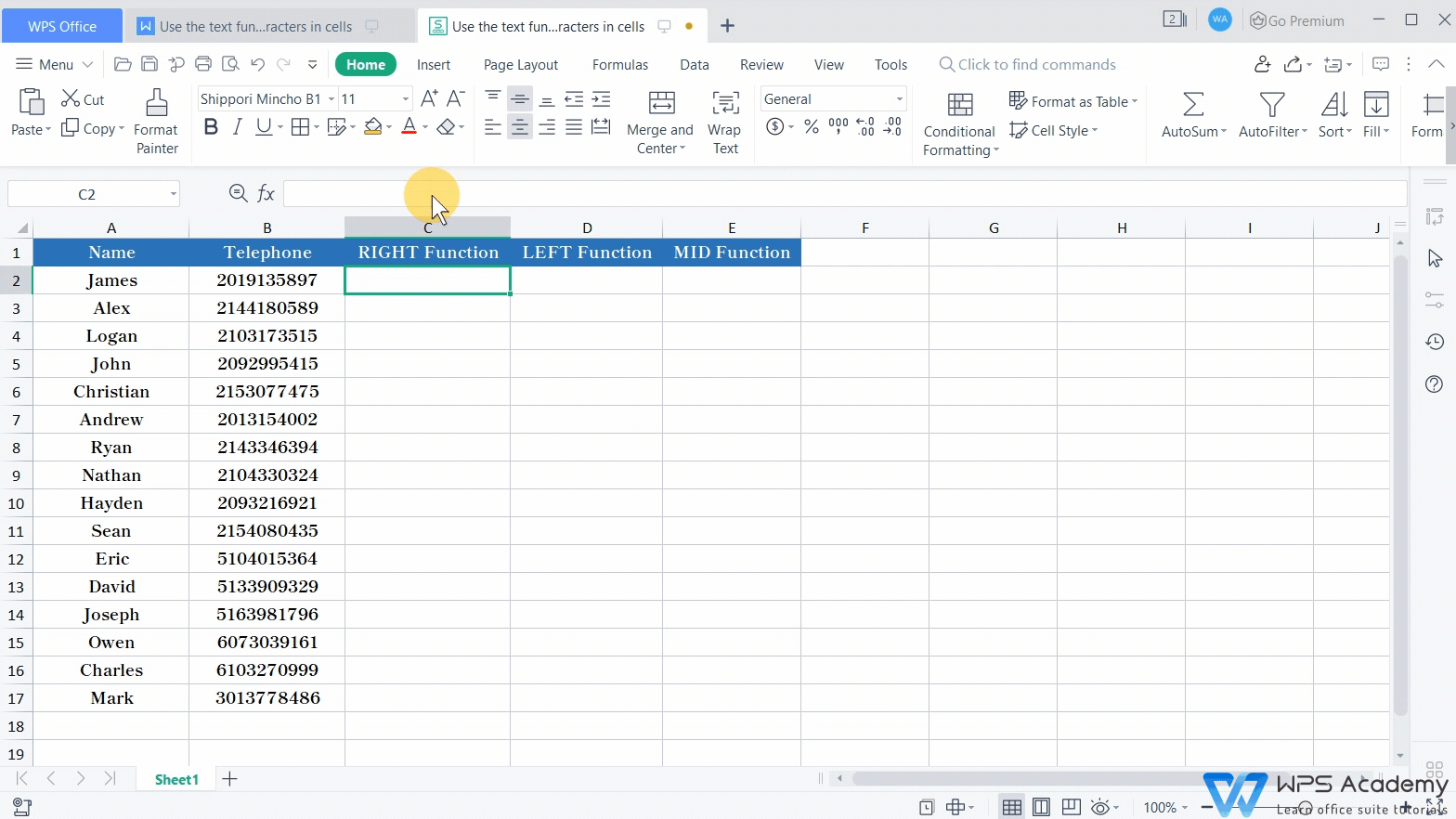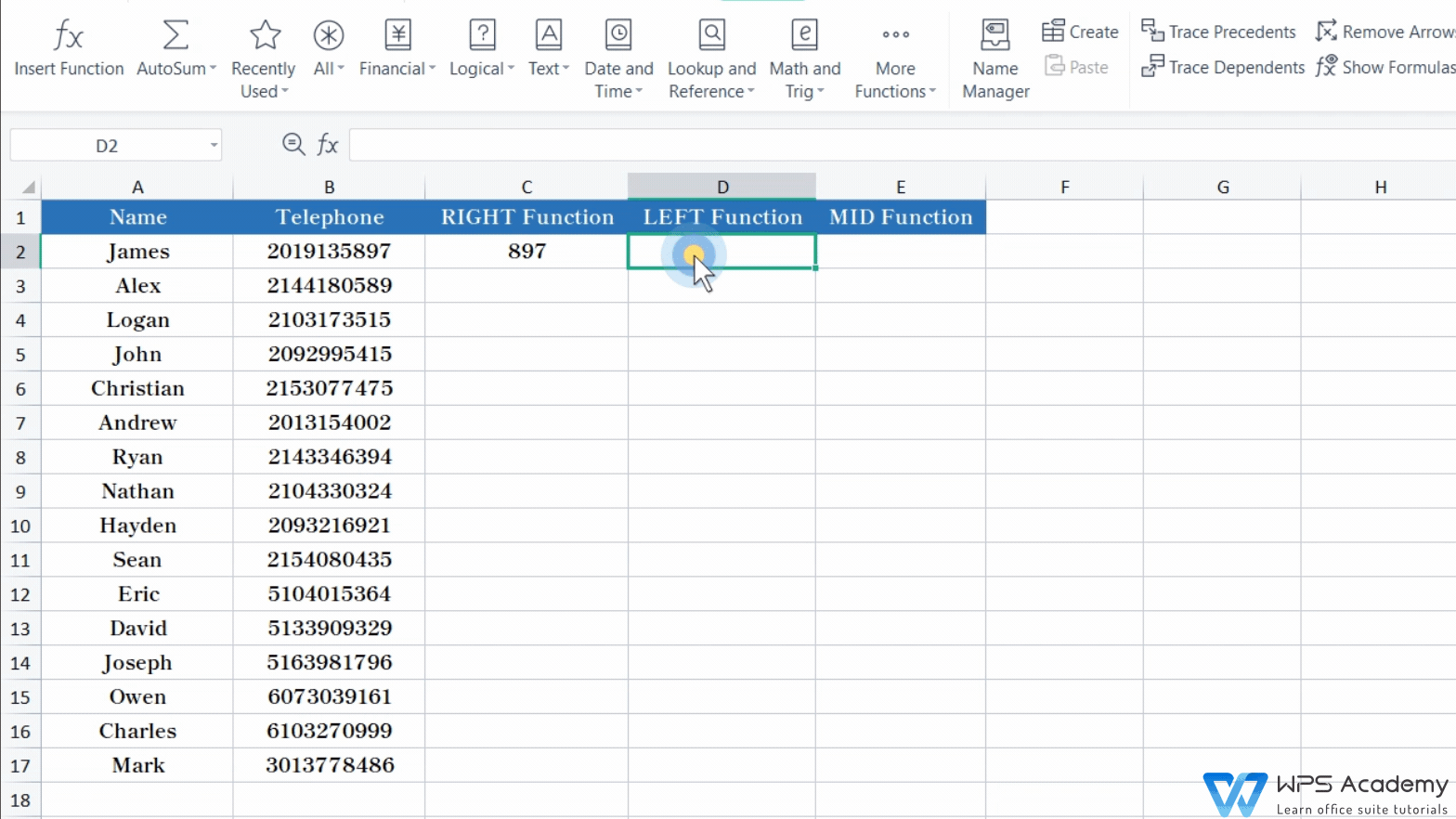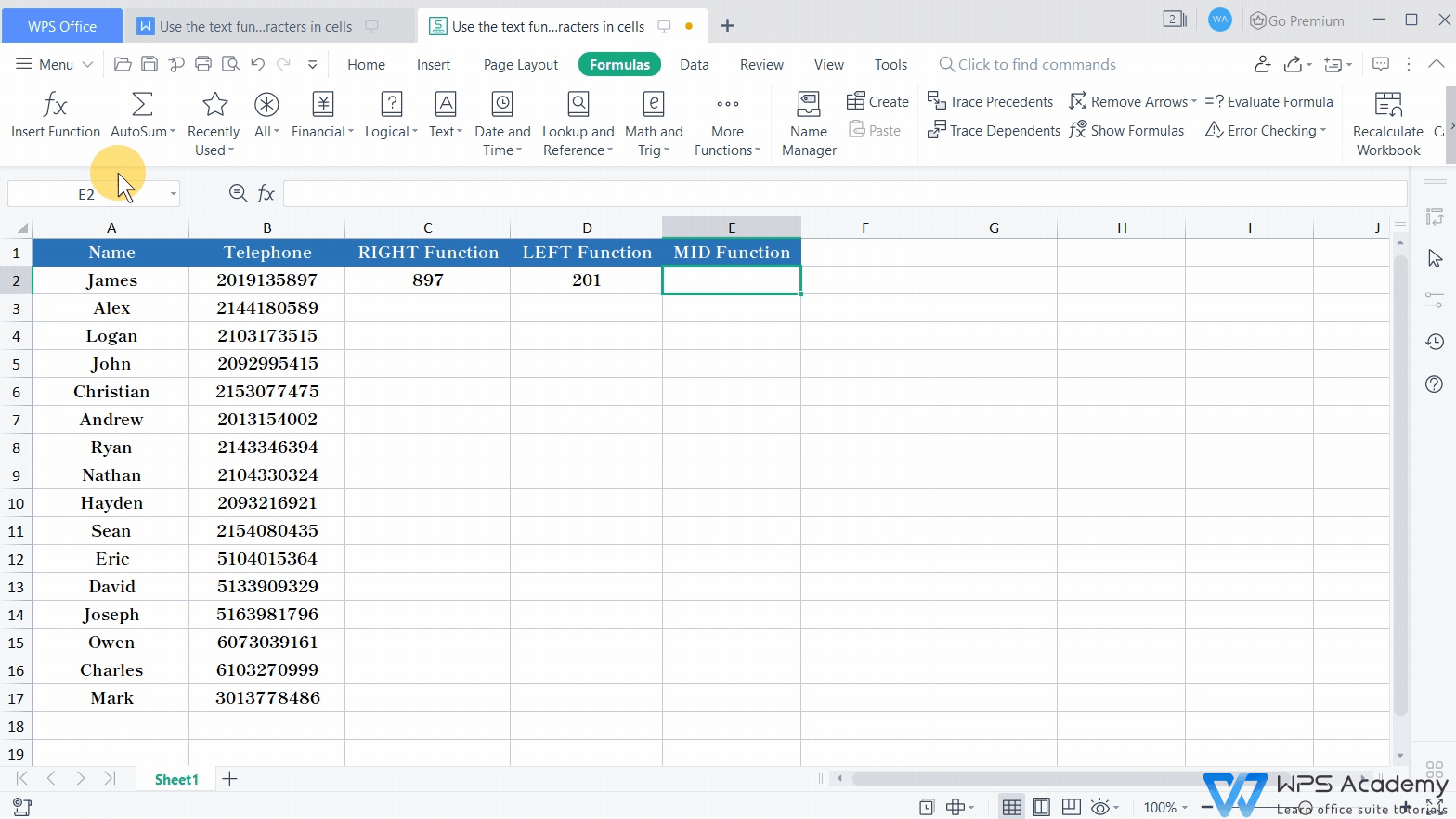# Use the text function to extract characters in cells

Uploaded time: October 8, 2021 Difficulty: Beginner# Use the text function to extract characters in cells

In this video, we'll introduce three practical functions in excel, which are the RIGHT, LEFT, and MID functions.

In this example, if we want to extract the first three numbers on the right, how can we realize it? The RIGHT function could help. It can extract characters within a cell, from the right to the left.

Here is the complete formula of the RIGHT function. RIGHT(text,num_chars)

The first parameter text refers to the specific text to be extracted. The second parameter num_chars refers to the character numbers that you want to extract. When the parameter is equal to 0, the function will return empty text. When a parameter is a negative number, the result will be #VALUE!.Select cell C2. Next, get into the Formulas tab, click the Insert Function button, and insert the RIGHT function. Enter B2 in the Text input box, and 3 in the Num_chars input box.The LEFT and RIGHT functions are similar. The only difference is the starting position. The RIGHT function starts to extract characters from the right to the left, while the LEFT function starts the extraction from the left to the right.

If we want to extract the first three numbers from the left in cell B2, how can we realize it? Get into the Formulas tab, click Insert Function, and insert the LEFT function. Enter B2 in the Text input box, and 3 in the Num_chars input box.Finally, it's an introduction on the MID function, which can extract the characters in the middle of the cell. In this example, what can we do to extract the four numbers 9135?

Select cell E2, and click Insert Function to insert the MID function.

The complete formula is:

MID(text,start_num,num_chars)

The first parameter text refers to the original text string. Here we enter B2 into the input box, which means we'll extract characters from cell B2. The second parameter start_num stands for the starting position of the extraction. Since we start to extract from the fourth character, we should enter 4 in the box. The third parameter num_chars means the number of characters to be extracted. Since we need to extract the four numbers 9135, which counts 4 characters in total, we should enter 4 in the box. Click OK to get the result.The three practical functions can help extract characters and text content from cells. Make your work easier with WPS Spreadsheet like Microsoft Excel. Did you get it?